## IF FUNCTION

The IF function returns one of two values depending on whether a specified expression evaluates to a Boolean value of TRUE or FALSE.

IF(test, then_value, otherwise_value)

test:  A logical expression. test can contain anything as long as the expression can be evaluated as a Boolean. If the expression evaluates to a number, 0 is considered to be FALSE, and any other number is considered to be TRUE.

then_value:  The value returned if the expression is TRUE. if-true can contain any value type. If omitted (comma but no value), IF will return 0.

otherwise_value:  An optional argument specifying the value returned if the expression is FALSE. if-false can contain any value type. If omitted (comma but no value), IF will return 0. If entirely omitted (no comma after if-false) and if-expression evaluates to FALSE, IF will return FALSE.

Usage Notes

(1) If the Boolean value of test is TRUE, the function returns the then_value; otherwise it returns the otherwise_value. Both then_value and otherwise_value can contain additional IF functions (nested IF functions).

(2) the then_value & otherwise_value is optional argument. if left it still the IF function works. if the test results in 1/0 or TRUE/FALSE the result displayed by the function is TRUE/FALSE depending on the test value evaluated.

What is Boolean:

A Boolean value is one that can be expressed in only one of two values, as either TRUE or as FALSE. A FALSE value also has the value of 0. In this case, any other numeric value is considered TRUE. Boolean values are mostly used in comparisons.

Lets understand the IF function with an examples

Exp:1

If i order up to 500 units of the a product, I pay 10.00 per unit. if order from 501 through 1200 units, i pay 9.00 per unit. if i order from 1201 through 2000 units, i pay 8 per unit. if i order more than 2000 units, i pay 7 per unit. How can i write formula that expresses the purchase cost as a function of the number of units purchased?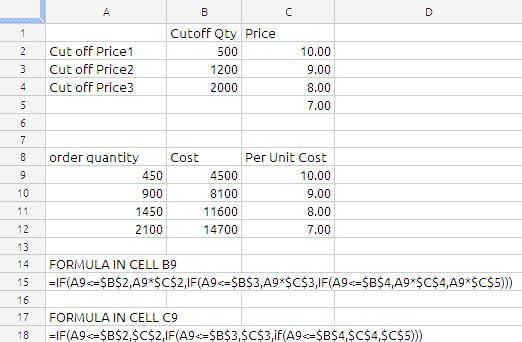we have used the nested IF conditions as shown in above image.

Exp 2:

If the value in Cell A1 is Red than value in the cell B1 will be 25 , if it is Blue then B1 has to be 50 and if A1 is Green then value in B1 has to be 75.

Solution: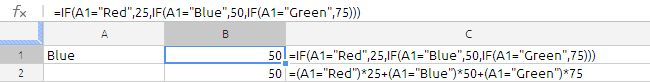in this case we have used two methods using IF function and using boolean algebra, Some time this type of situation using of boolean algebra will much more understandable and it is not constrained by IF function limitations.

This simple comparison will result in a value of TRUE if A2 has “Red” in it (just to be clear: the string of letters, not the background color). If there is anything else or even nothing in A2, then the result will be FALSE. True and False are actual values in the spreadsheet. If you were to enter this formula in some cell, you would see the word TRUE or the word FALSE in that cell, depending on what was in A2.

the spreadsheet is evaluated as shown below:

=(False)*25 + (True)*50 + (False)*75

= (0)*25+(1)*50+(0)*75

=0+50+0

=50

the other method of evaluating this is by use of CHOOSE function, the formula will be

=choose(MATCH(A1,F1:F3,0),25,50,75)

where F1 to F3 will hold Red,Blue, Green

we can also use the vlookup

The IF function has endless uses so we cannot document everything in one article. so i am concluding this article.

## Maximum Based on Multiple Criteria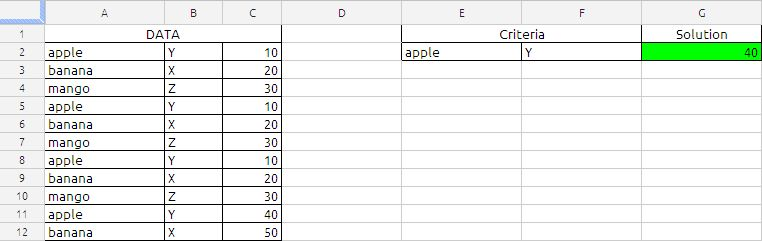The Formula in cell G2 is
=ArrayFormula(MAX(IF(A2:A12=E2,IF(B2:B12=F2,C2:C12))))

the above formula will return the maximum value in cell C2:C12 corresponding to criteria specified in the cell E2 and F2.

the formula will narrows down using the conditions in the IF function first A2:A12 and then B2:B12. If the both conditions are met the resultant will be value in cell C2:C12

Which will be MAX {10,10,10,40}

based on the sample data the result will be 40.

## unique random number between 1,10 in single array formula

The Randbetween function may generate duplicate value. if we used the formula

=Randbetween(1,10) it will generate random numbers between 1 and 10, if we applied the formula in ten cells there is a possibility the formula may generate duplicate value in the ten cells. To avoid this you can use the following formula

=ARRAYFORMULA(LARGE(ROW(\$1:\$10)*NOT(COUNTIF(\$A\$1:A1, ROW(\$1:\$10))), RANDBETWEEN(1,11-ROW(A1))))

Copy cell A2 and paste down as far as needed.

### Explaining the alternative array formula in cell A2

Step 1 – Create an array
ROW(\$1:\$10) creates this array {1, 2, 3, 4, 5, 6, 7, 8, 9, 10}

Step 2 – Create a criterion to avoid duplicate numbers
COUNTIF(\$A\$1:A1, ROW(\$1:\$10)) makes sure no duplicate numbers are created. The formula has both absolute and relative cell references (\$A\$1:A1). When the formula are copied down to cell A3 the cell reference changes to \$A\$1:A2. The value in cell A2 can´t be randomly selected again.
In cell A2, COUNTIF(\$A\$1:A1, ROW(\$1:\$10)) creates this array: {0, 0, 0, 0, 0, 0, 0, 0, 0, 0}

Step 3 – Create a new dynamic array
ROW(\$1:\$10)*NOT(COUNTIF(\$A\$1:A1, ROW(\$1:\$10))) creates this array in cell A2: {1, 2, 3, 4, 5, 6, 7, 8, 9, 10}
If the array formula  randomly selects the number 2 in cell A2, the formula in cell A3 creates this array: {1, 0, 3, 4, 5, 6, 7, 8, 9, 10}
Number 2 can´t be selected anymore.

Step 4 – Calculate the number range in Randbetween(bottom, top)
The bottom value is always 1. The top value changes depending on current cell.
In cell A2 the top value is 10.
In cell A3 the top value is 9
and so on..
Formula in cell A2: 11-ROW(A1) equals 10. (11-1=10)
Formula in cell A3: 11-ROW(A2) equals 9. (11-2=9)
and so on..

Step 5 – Create a random number
=LARGE(ROW(\$1:\$10)*NOT(COUNTIF(\$A\$1:A1, ROW(\$1:\$10))), RANDBETWEEN(1,11-ROW(A1)))
RANDBETWEEN(1,11-ROW(A1))
becomes
RANDBETWEEN(1,11-1)
becomes
RANDBETWEEN(1,10)
and returns a random number between 1 and 10.

Step 6 – Select a random number in array
=LARGE(ROW(\$1:\$10)*NOT(COUNTIF(\$A\$1:A1, ROW(\$1:\$10))), RANDBETWEEN(1,11-ROW(A1)))
becomes
=LARGE({1, 2, 3, 4, 5, 6, 7, 8, 9, 10}, RANDBETWEEN(1,10))
becomes
=LARGE({1, 2, 3, 4, 5, 6, 7, 8, 9, 10}, random_number) and returns a random number between 1 and 10.

We are looking into some more Weekday formula in addition to my earlier blog post about Weekday function.

In this example we are identifying  the first and last weekdays using the Weekday, Month, Date & MOD function in Google Docs Speadsheets.

The Chosen date is in cell B1,

the link to my earlier Weekday function

## SMALL

The SMALL function returns the nth-smallest value within a range. The smallest value is ranked number 1.

The Syntax of the function is

SMALL(data, rank_c)

data:  A collection of values. data may be a number,date,duration,set is a collection containing number, date, or duration values. All values must be of the same type.

rank_c:  A number representing the size ranking of the value you want to retrieve. ranking is a number value and must be in the range of 1 to the number of values in the collection.

Notes:A ranking of 1 retrieves the smallest number in the collection, 2 the second-smallest, and so on. Values included in the collection that are of the same size are ranked together, but impact the outcome.

We will try to understand the function with an example.

The sample data set is follows: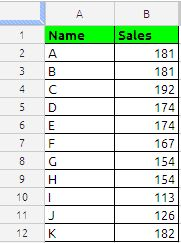if we want to identify the small three numbers of above data set  conventional way is as follows: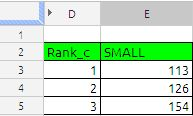The formula in cell E3 is

=SMALL(\$B\$2:\$B\$12,D3) and drag it down till E5 we will get the 1st smallest 2nd smallest and 3rd smallest.

other way to extract largest 3 value with more robust formula is with use of ROWS function. the formula will be

=SMALL(\$B\$2:\$B\$11,ROWS(E\$3:E3)) and drag it down here we do not need a helped column D where argument rank_c.

Google Docs way of writing the formula is

=ArrayFormula(if(len(D3:D5);SMALL(B2:B12,D3:D5);iferror(1/0)))

In the above formula you do not require to drag any thing the formula will automatically put 1st, 2nd and 3rd smallest value in cell E3 to E5.

if we want to largest numbers across the columns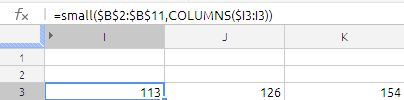The formula in cell I3 is

=SMALL(\$B\$2:\$B\$11,COLUMNS(\$I3:I3)) just drag it across.

if we want the sum of large three numbers in single cell,  formula will be as follows:

=sum(large(B2:B12;{1,2,3}))

which is nothing but sum of  113+126+154 = 393.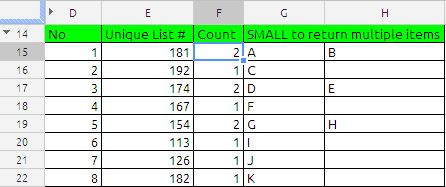With the use to small function we can extract data of duplicate records as shown in the above figure.

the formula in cell E15 is

=UNIQUE(B2:B12)

the formula in cell F15 is

=COUNTIF(\$B\$2:\$B\$12,E15) and drag it down

and the formula in cell G15 is

=IF(COLUMNS(\$G15:G15)>\$F15,””,INDEX(\$A\$2:\$A\$12,SMALL(IF(\$B\$2:\$B\$12=\$E15,ROW(\$B\$2:\$B\$12)-ROW(\$B\$2)+1),COLUMNS(\$G15:G15)))) drag the formula down and across.

the formula in cell G15 can be modified with filter formula in google docs way of writing

=transpose(FILTER(A:A,B:B=E15)) and drag it down.